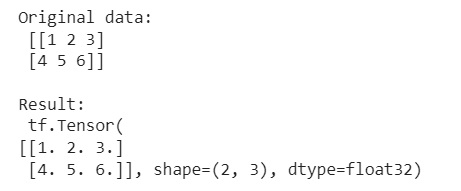# Convert NumPy arrays to standard TensorFlow format

Learn, how to convert NumPy arrays to standard TensorFlow format in Python? By Pranit Sharma Last updated : March 30, 2023

Suppose that we are given a numpy array that contains an image. We know that a picture containing pixels arranges as a matrix hence we can import the pixels of this image as a matrix.

We need to load these files into TensorFlow to perform certain machine-learning operations on it.

## Converting NumPy array into TensorFlow format

To convert the numpy array into TensorFlow format, we can simply use tf.convert_to_tensor() after importing the TensorFlow library.

Let us understand with the help of an example,

## Python code to convert NumPy array into TensorFlow format

```# Import numpy
import numpy as np

# Import tensorflow
import tensorflow as tf

# Creating a numpy array
arr = np.array([[1,2,3],[4,5,6]])

# Display original data
print("Original data:\n",arr,"\n")

# Converting array to TensorFLow
res = tf.convert_to_tensor(arr, np.float32)

# Display result
print("Result:\n",res,"\n")
```

### Output